# Coordinate systems in ggplot2

Coordinate systems in ggplot2 can be divided into two categories: linear (`coord_cartesian`, `coord_fixed`, `coord_flip`) and non-linear (`coord_trans`, `coord_polar`, `coord_quickmap`, `coord_map`) coordinate systems. These systems will be reviewed in this tutorial.

## Cartesian coordiantes with `coord_cartesian`

By default, ggplot2 charts have cartesian coordinate. However, the `coord_cartesian` function is very useful for zooming the plots, because if you use `scale_x_continuous` or `scale_y_continuous` the data will change the underlying data and hence the calculated stats, as shown below.

Default cartesian coordinates

``````# install.packages("ggplot2")
library(ggplot2)

# Data
set.seed(4)
df <- data.frame(x = rnorm(200),
y = rnorm(200))

p <- ggplot(df, aes(x = x, y = y)) +
geom_point() +
geom_smooth()

p``````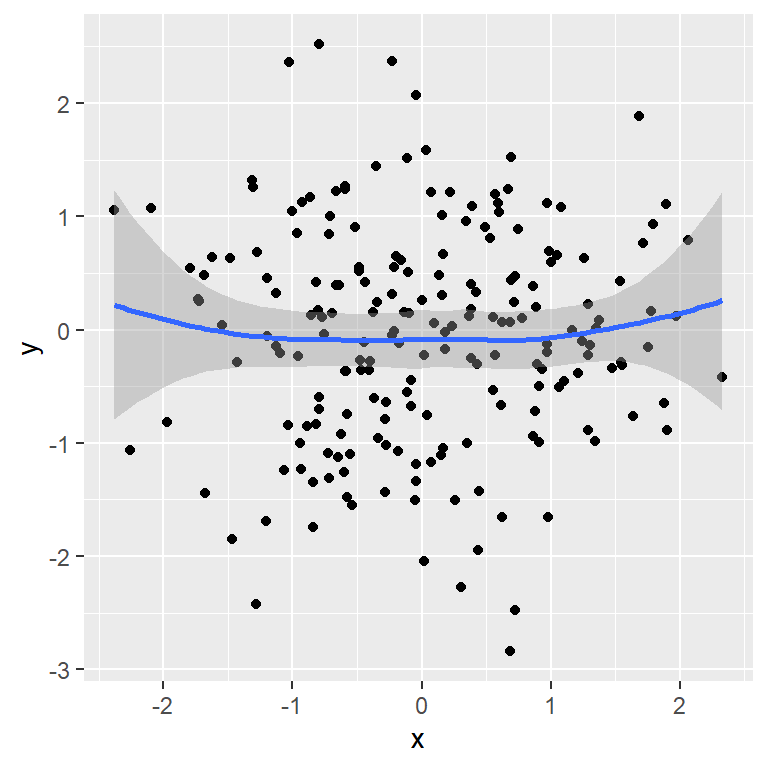Using `scale_x_continuous` for zooming modifies the underlying data and the smooth estimation.

``````# install.packages("ggplot2")
library(ggplot2)

# Data
set.seed(4)
df <- data.frame(x = rnorm(200),
y = rnorm(200))

p <- ggplot(df, aes(x = x, y = y)) +
geom_point() +
geom_smooth()

p + scale_x_continuous(limits = c(-1, 1))``````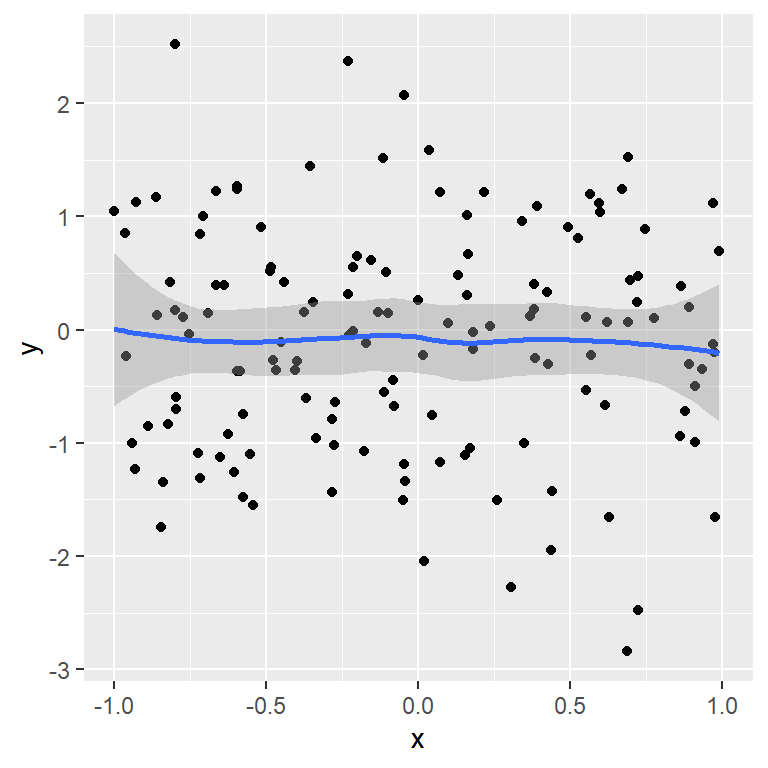However, if you use `coord_cartesian` you can set the `xlim` and `ylim` without modifying the original estimations and just zooming in or out.

``````# install.packages("ggplot2")
library(ggplot2)

# Data
set.seed(4)
df <- data.frame(x = rnorm(200),
y = rnorm(200))

p <- ggplot(df, aes(x = x, y = y)) +
geom_point() +
geom_smooth()

p + coord_cartesian(xlim = c(-1, 1))``````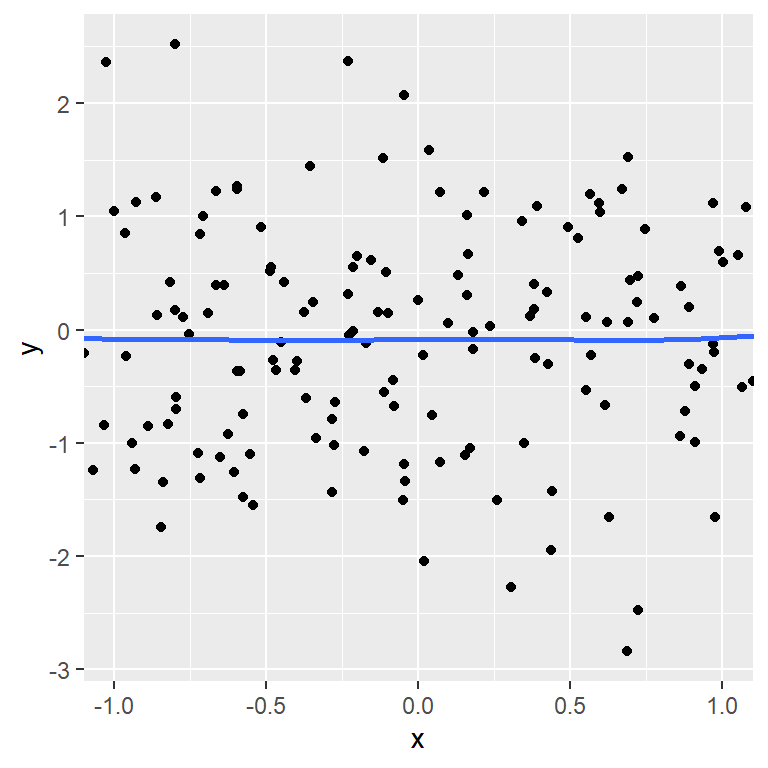## Fixed coordinates with `coord_fixed`

The `coord_fixed` function is very useful in case you want a fixed aspect ratio for your plot regardless the size of the plotting device. By default, one unit along the X axis will be the same unit along the Y axis, so the scales of the axes will be equal. You can use the `ratio` argument to specify the desired aspect ratio expressed as `y/x`.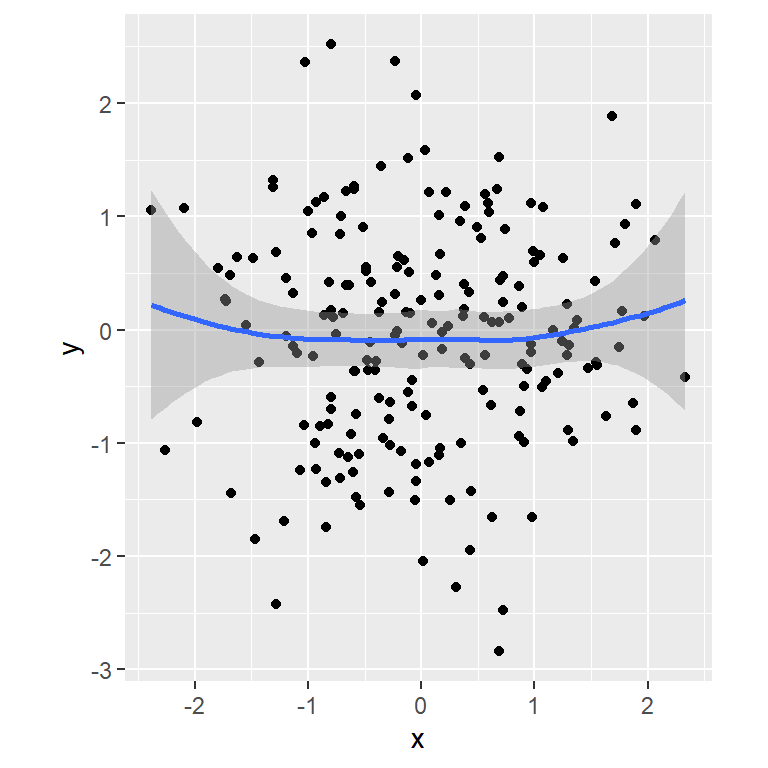``````# install.packages("ggplot2")
library(ggplot2)

# Data
set.seed(4)
df <- data.frame(x = rnorm(200),
y = rnorm(200))

p <- ggplot(df, aes(x = x, y = y)) +
geom_point() +
geom_smooth()

p + coord_fixed()``````

## Flipping the axes with `coord_flip`

The `coord_flip` function rotates the axes in ggplot2, so if you have a vertical plot you can create the horizontal version and viceversa. This is specially useful for box plots, violin plots, …

Default

``````# install.packages("ggplot2")
library(ggplot2)

# Data
set.seed(4)
df <- data.frame(x = rnorm(200))

p <- ggplot(df, aes(x = x, y = "")) +
geom_boxplot()

p``````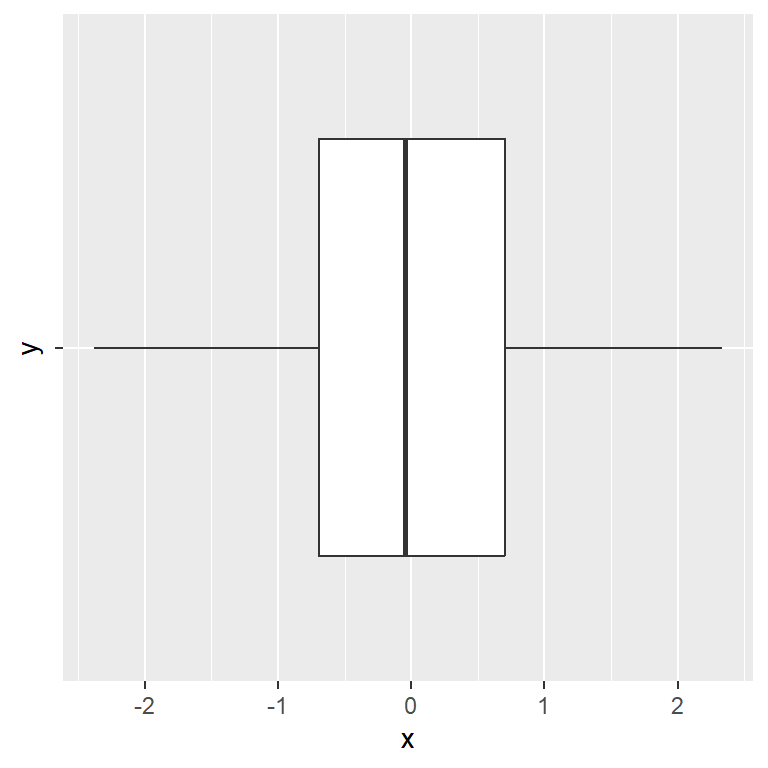Flipped axes

``````# install.packages("ggplot2")
library(ggplot2)

# Data
set.seed(4)
df <- data.frame(x = rnorm(200))

p <- ggplot(df, aes(x = x, y = "")) +
geom_boxplot()

p + coord_flip()``````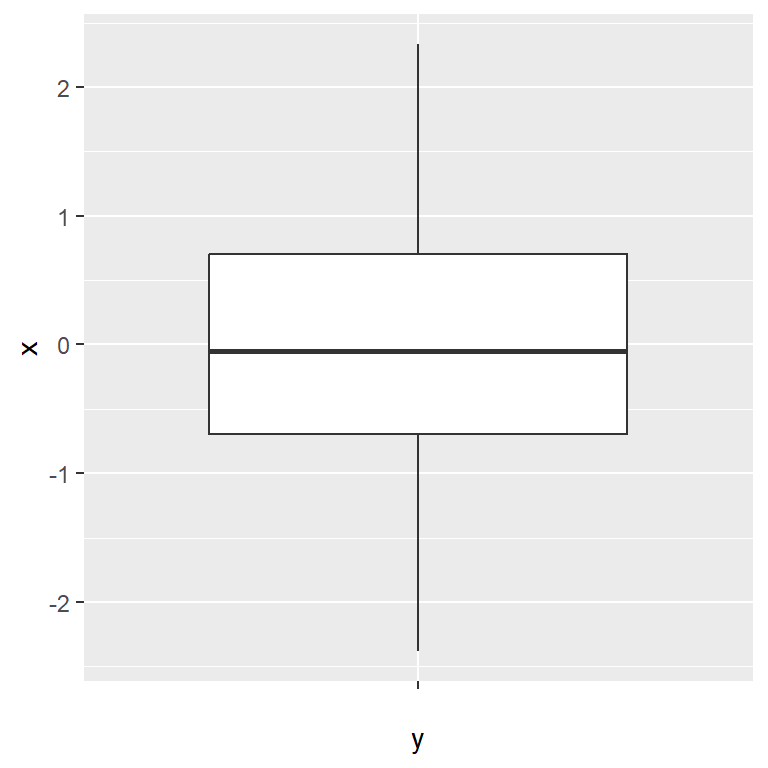## Transformations with `coord_trans`

The `coord_trans` function creates transformed cartesian coordinate systems. Note that using this functions is not the same as transforming the scale, due to when using `coord_trans` the transformation occurs after statistics, affecting the visual appearance of geoms. Consider the following sample plot: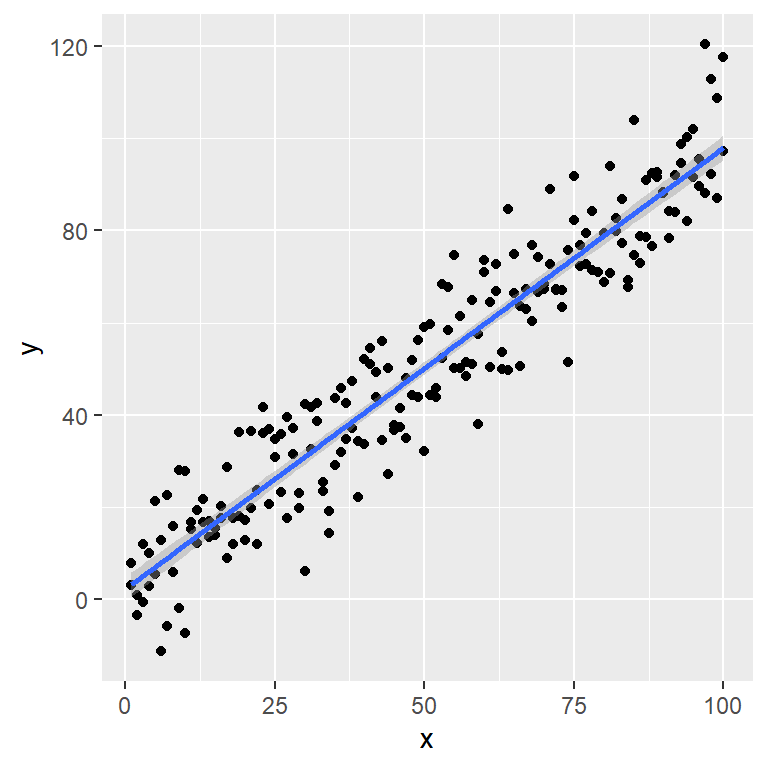``````# install.packages("ggplot2")
library(ggplot2)

# Data
set.seed(4)
df <- data.frame(x = 1:100,
y = 1:100 + rnorm(200, sd = 10))

p <- ggplot(df, aes(x = x, y = y)) +
geom_point() +
geom_smooth(method = "lm")

p``````

In the following example we are transforming the X-axis (`x` argument) with the `log` function, so the linear estimate will become a curve. Recall that you can pass any function to the axes as long as it makes sense.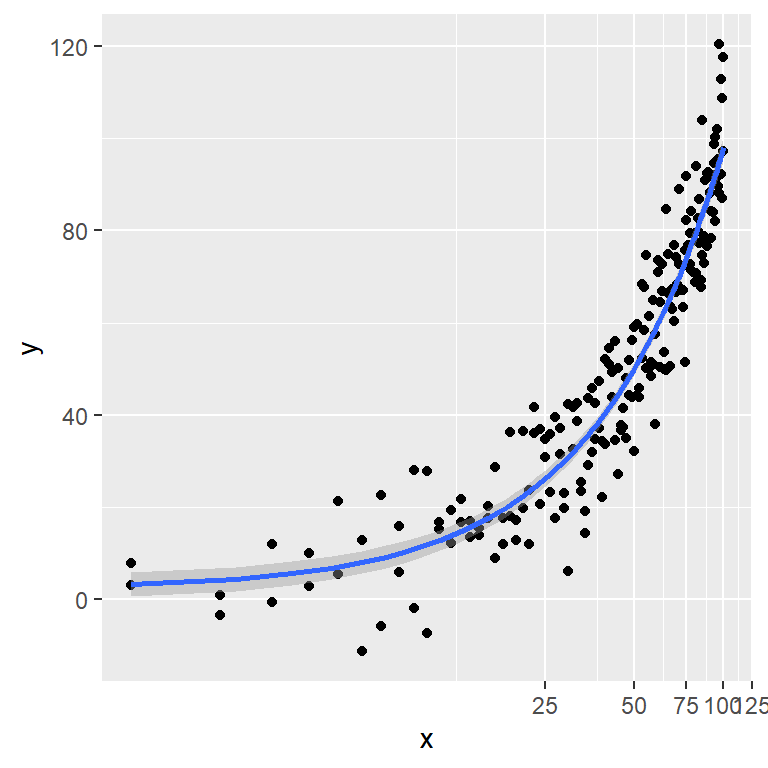``````# install.packages("ggplot2")
library(ggplot2)

# Data
set.seed(4)
df <- data.frame(x = 1:100,
y = 1:100 + rnorm(200, sd = 10))

p <- ggplot(df, aes(x = x, y = y)) +
geom_point() +
geom_smooth(method = "lm")

p + coord_trans(x = "log")``````

## Polar coordinates with `coord_polar`

The polar coordinates can be created using `coord_polar`. This type of coordinates are commonly used for pie charts (which are stacked bar charts in polar coordinates), wind roses, radar charts, bullseye charts, …

Note that by default the angle is mapped to the `x` variable, but you can set `theta = "y"` to map the angle to the `y` variable. You can also modify the direction of the plot with the `direction` argument (set `-1` for anticlockwise). See the examples below for clarification.

``````# install.packages("ggplot2")
library(ggplot2)

# Data
set.seed(4)
df <- data.frame(x = 1:10,
y = sample(1:10))

p <- ggplot(df, aes(x = x, y = y, fill = y)) +
geom_bar(stat = "identity", color = "white",
lwd = 1, show.legend = FALSE)

p + coord_polar()``````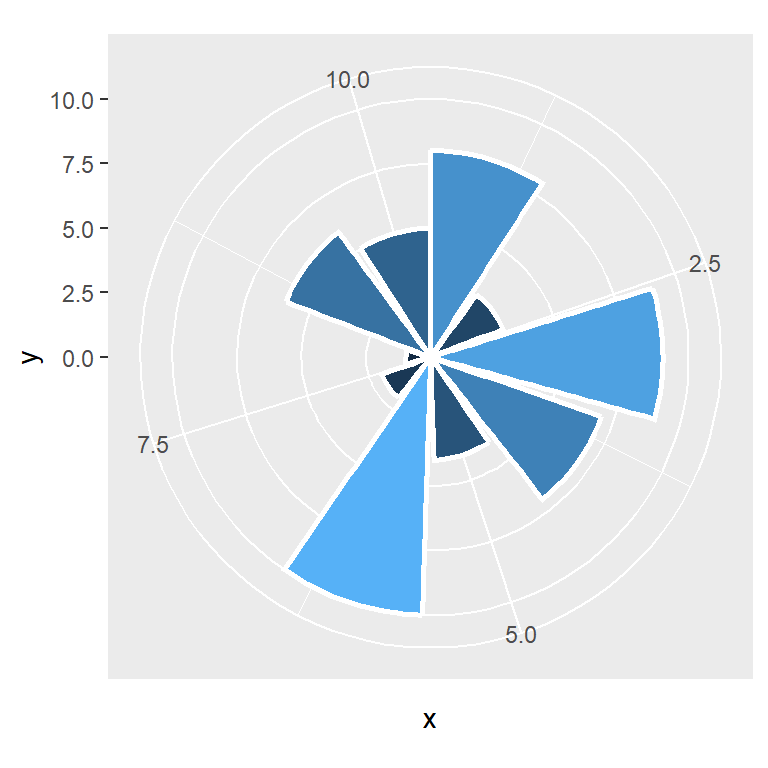``````# install.packages("ggplot2")
library(ggplot2)

# Data
set.seed(4)
df <- data.frame(x = 1:10,
y = sample(1:10))

p <- ggplot(df, aes(x = x, y = y, fill = y)) +
geom_bar(stat = "identity", color = "white",
lwd = 1, show.legend = FALSE)

p + coord_polar(theta = "y")``````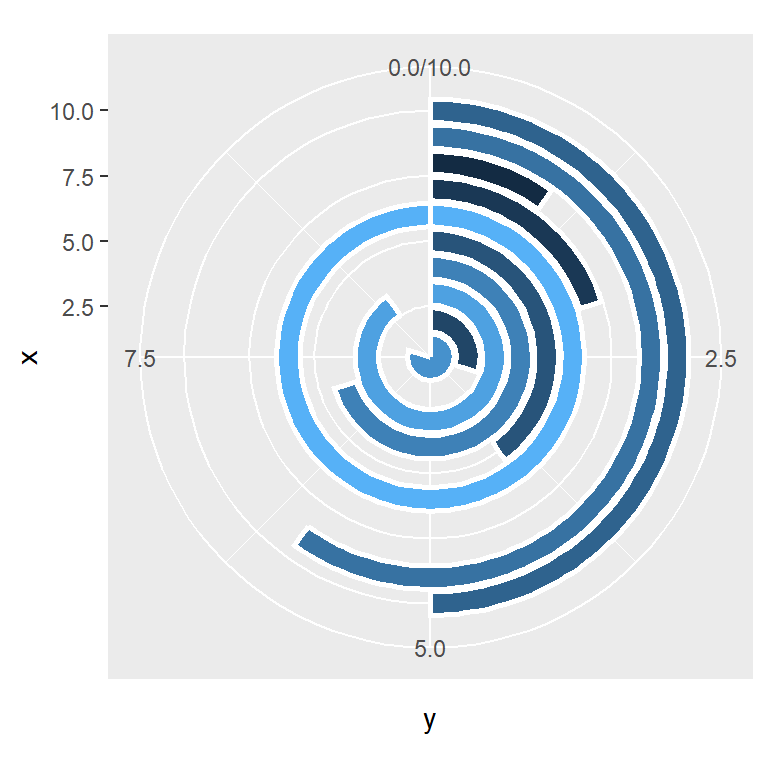## Map projections with `coord_quickmap` and `coord_map`

The last system coordinates are related to map projections. You will need to have the `mapproj` package installed in your computer for applying the projections.

On the one hand, the `coord_map` function requires considerable computation, but will approximate the projection as much as possible. Note that there are lots of different projections available. Type `?mapproj::mapproject` for the full list.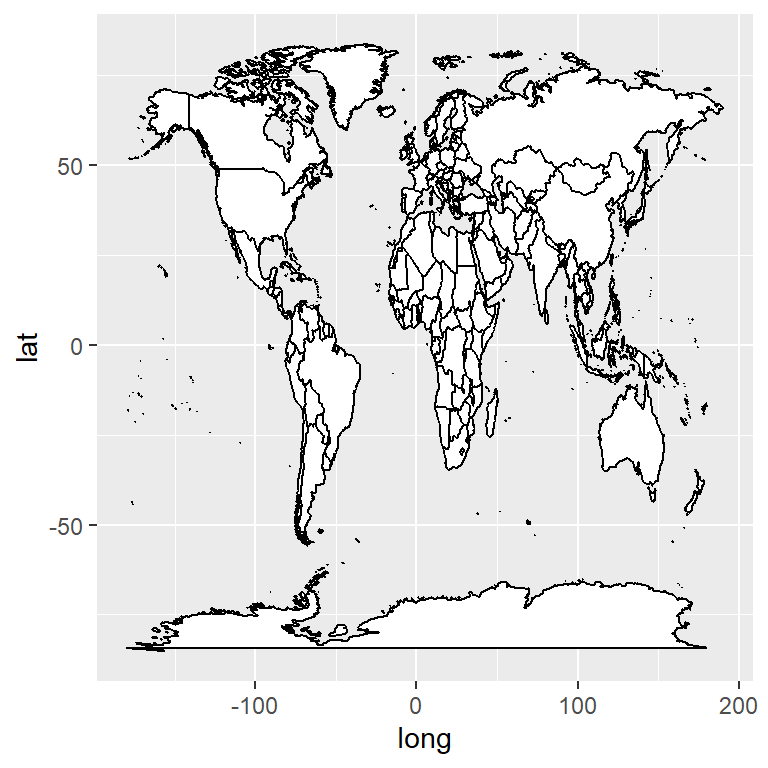Default map

``````# install.packages("ggplot2")
library(ggplot2)
# install.packages("mapproj")

p <- ggplot(map_data("world"),
aes(long, lat, group = group)) +
geom_polygon(fill = "white", colour = 1)

p``````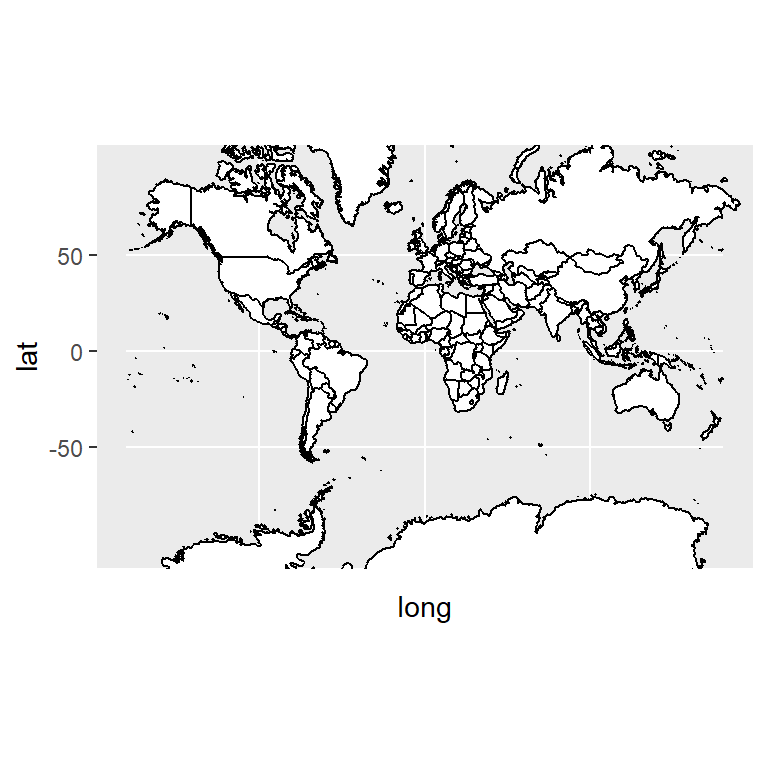Mercator projection

The default projection is the well-known Mercator projection. Note that we modified the limits of the X-axis due to the function produces some unwanted horizontal lines. If you want to create this plot using `coord_sf` from `sf` package is a better solution.

``````# install.packages("ggplot2")
library(ggplot2)
# install.packages("mapproj")

p <- ggplot(map_data("world"),
aes(long, lat, group = group)) +
geom_polygon(fill = "white", colour = 1)

p + coord_map(xlim = c(-180, 180))
# p + sf::coord_sf() # Better solution``````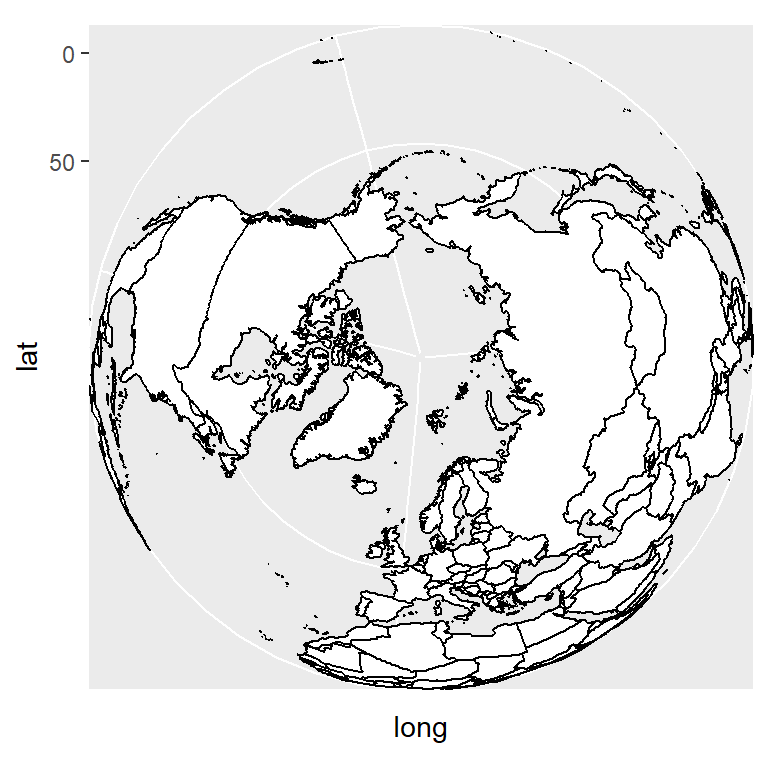Orthographic projection

``````# install.packages("ggplot2")
library(ggplot2)
# install.packages("mapproj")

p <- ggplot(map_data("world"),
aes(long, lat, group = group)) +
geom_polygon(fill = "white", colour = 1)

p + coord_map("orthographic")``````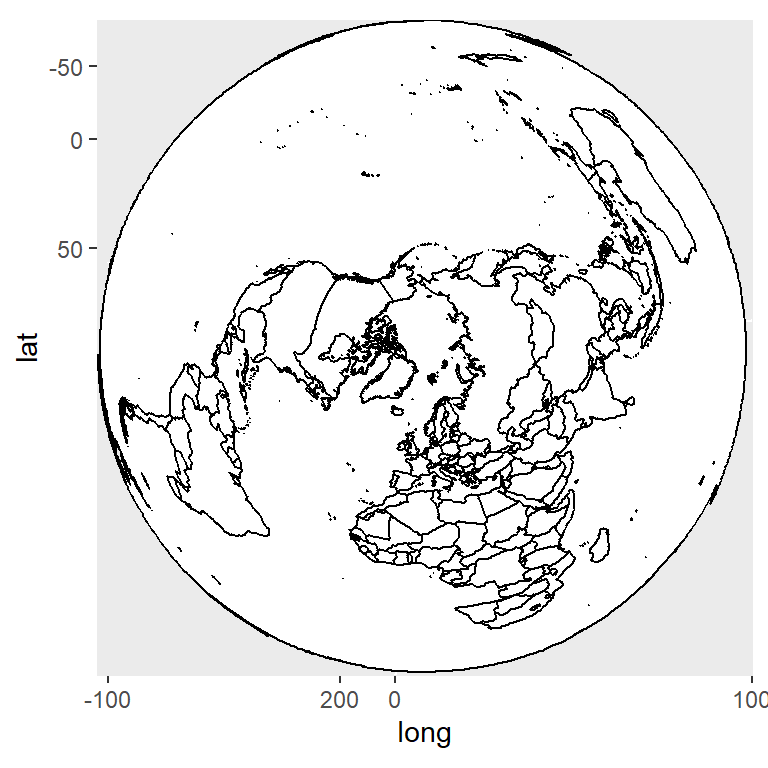Fish eye

``````# install.packages("ggplot2")
library(ggplot2)
# install.packages("mapproj")

p <- ggplot(map_data("world"),
aes(long, lat, group = group)) +
geom_polygon(fill = "white", colour = 1)

p + coord_map("fisheye",
n = 4) # Refractive index``````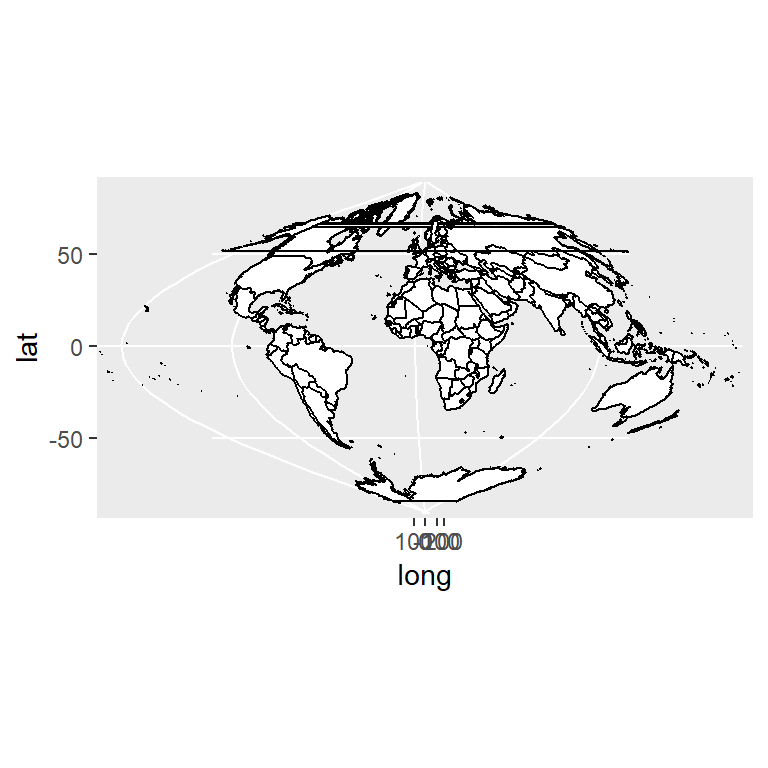Sinusoidal

``````# install.packages("ggplot2")
library(ggplot2)
# install.packages("mapproj")

p <- ggplot(map_data("world"),
aes(long, lat, group = group)) +
geom_polygon(fill = "white", colour = 1)

p + coord_map("sinusoidal")``````

On the other hand, `coord_quickmap` also fixes the projection of a map, as shown below, but this function is a quick approximation for preserving straight lines which works best for small areas closer to the equator. Note that you can also apply any desired projection with the `projection` argument of the function, as in the previous examples.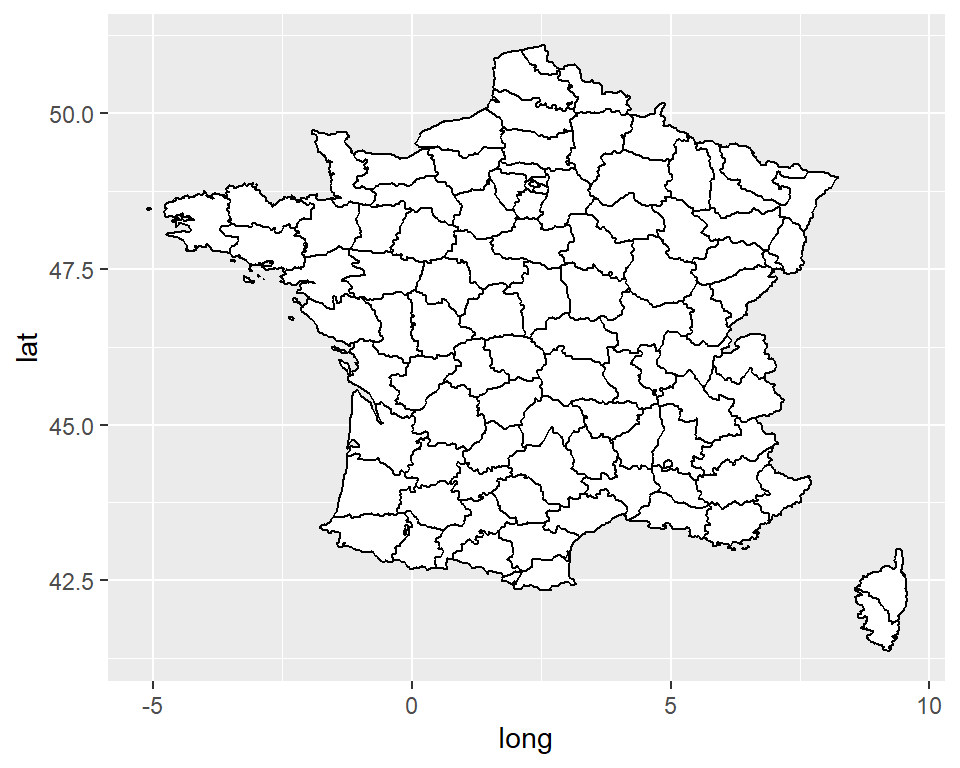Default map

``````# install.packages("ggplot2")
library(ggplot2)

p <- ggplot(map_data("france"),
aes(long, lat, group = group)) +
geom_polygon(fill = "white", colour = 1)

p``````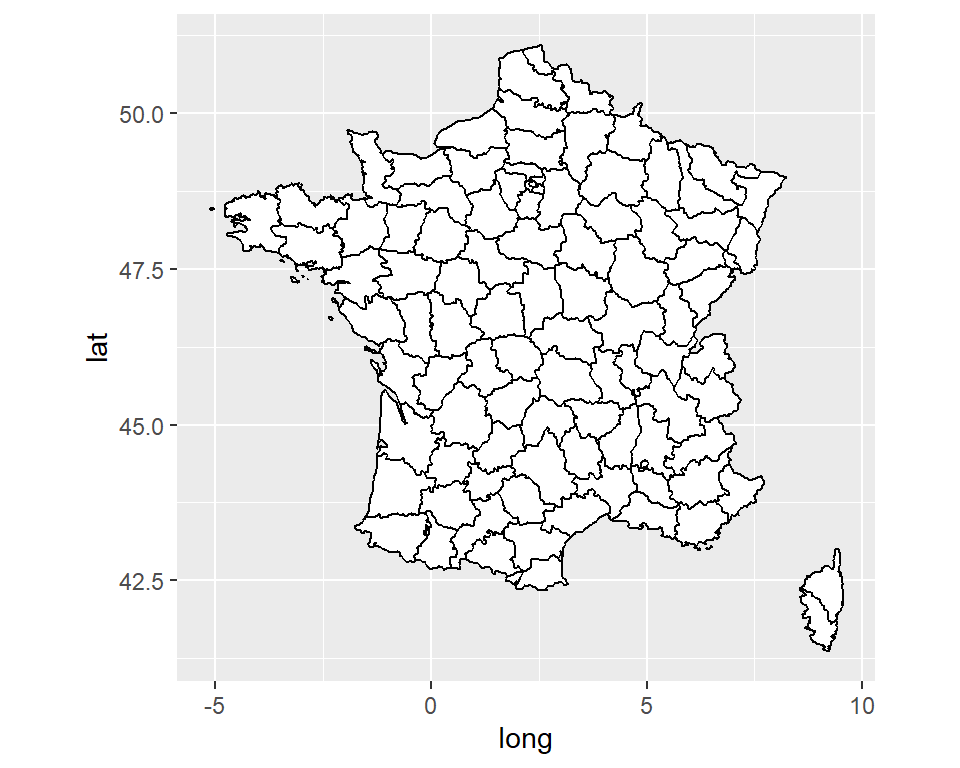Fixing the projection

``````# install.packages("ggplot2")
library(ggplot2)

p <- ggplot(map_data("france"),
aes(long, lat, group = group)) +
geom_polygon(fill = "white", colour = 1)

p + coord_quickmap()``````